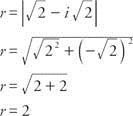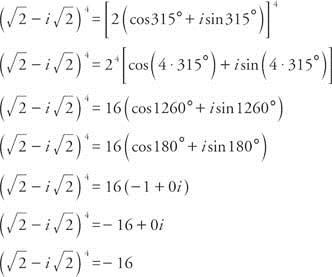×#### Thank you for registering.

One of our academic counsellors will contact you within 1 working day.

Click to Chat

1800-1023-196

+91-120-4616500

CART 0

• 0

MY CART (5)

Use Coupon: CART20 and get 20% off on all online Study Material

ITEM
DETAILS
MRP
DISCOUNT
FINAL PRICE
Total Price: Rs.

There are no items in this cart.
Continue Shopping```Demoivre's Theorem: The Theorem can be Stated in two Forms:

Demoivre’s theorem is an important part of the complex numbers. It is a simple topic and fetches a good amount of questions in the Mathematics portion of the JEE exam. We first start by stating the theorem and then proceed towards the applications:Case I

Statement:

If n is any integer, then

(i) (cos θ + i sin θ)n = cos nθ + i sin nθ

(ii) (cos θ1 + i sin θ1) = (cos θ2 + i sin θ2) ......... (cos θn + i sin θn)

= cos θ1 + θ2 + θ3 .................. + θn) + i sin (θ1 + θ2 + ..............  θ3)

Case II

Statement:

If p, q ε and q ≠ 0, then (cos θ + i sin θ)p/q = cos((2kπ + pq)/q) + isin((2kπ+pq/q)  where k = 0,1,2,3,.....,q-1

Note:

Continued product of the roots of a complex quantity should be determined using theory of equations.

Derivation: The Demoivre’s formula can be derived from the Euler’s formula:

eix = cos x +i sin x

The exponential law for integral powers givesThen by Euler’s Formula

e i(nx) = cos (nx) + i sin (nx)

A more elementary motivation of the theorem comes from calculating

(cos + isin x)2 = cos2x + 2i sin x cosx –sin 2x

= (cos2x – sin2x ) + i(2sinx cosx ) = cos (2x) +i sin(2x)

where the last equality follows from the trigonometric identities.

Hence, this proves the result for n=2

Failure for non-integer powers

In general, Demoivre’s formula does not hold for non-integer powers. Many different values are possible for the non-integer powers. But there is one generalization to this that the right hand side expression is one possible value of the power.

As seen in the derivation above, the Demoivre’s theorem involves a complex number to the power n. But in case the power is not an integer, the result is multi-valued.  For instance, consider the case when n=1/2 then,

For x = 0 the formula gives 1½ = 1

For x = 2π the formula gives 1½ = −1.

Since the angles 0 and 2π are the same this would give two different values for the same expression. The values 1 and −1 are however both square roots of 1.

These issues are not generally experienced wi9th the Euler’s formula since its different exponent powers are not generally considered. Euler's formula involves a complex power of a positive real number and this always has a defined value. The corresponding expressions are:

eiθ =1

eiπ= -1.

Applications

Demoivre’s Theorem has numerous applications. Its major application is in finding the nth roots of a complex number. This application does not strictly use Demoivre’s formula as the power is not an integer. However considering the right hand side to the power of n will, in each case, give the same value on the left-hand side.

Now if we write the complex number z in the polar form then,

z = r(cos x + isin x)

Then z1/n = [r (cos x +i sinx )]1/n = r 1/n [ cos ( x+2kπ/n) + i sin (x+2kπ/n)]

Here k is an integer. To get the n different roots of z one only needs to consider values of k from 0 to n − 1.

The following video will provide you more clarity

Example: Write (√2-i√2)4 in the form a + bi.Since cos α = √2/2 and sin α = - √2/2, α must be in the fourth quadrant and α = 315°. Therefore,You may also consult the Model Papers to get an idea about the types of questions asked.

Example:  Find the value of x from

x4 − x³ + x² − x + 1 = 0.

Solution: Seeing the given expression it can be judged that it is a part of the expansion of (x5+1).

And we know that
x5 + 1 = (x + 1) (x4 − x³ + x² − x + 1)
(x4 − x³ + x² − x + 1) = (x5 + 1) / (x + 1), for x ≠ −1
x4 − x³ + x² − x + 1 = 0
(x5 + 1) / (x + 1) = 0, for x ≠ −1
x5 + 1 = 0, for x ≠ −1
x5 = −1, for x ≠ −1

We can find values for x using De Moivre's theorem given x5 = −1
This will actually give us 5 values for x, but we exclude x = −1
x5 = −1 = cos(180) + i sin(180)
x = cos(180/5 + 360/5 k) + i sin(180/5 + 360/5 k), k = 0, 1, 2, 3, 4
x = cos(36+72k) + i sin(36+72k)

Now k = 2 will give x = −1, which is not a solution, so we ignore it.
x = cos(36) + i sin(36)
x = cos(108) + i sin(108)
x = cos(252) + i sin(252)
x = cos(324) + i sin(324)

Example: Compute (3+3i)5.

Solution: We first convert the given term in exponential form and then by using the Demoivre’s theorem we can get the required answer.

So consider the term (3+3i) and in this case r = √9+9 = 3√2.

tan θ= 3/3  so Arg z= π/4.

3+3i = 3√2 ei π/4

(3+3i)5 = (3√2)5 e i5π /4

= 972√ 2 (cos (5π/4) +isin (5π/4))

=   972√ 2 (-√2/2 – √2/2 i)

= -972-972i.

To read more, Buy study materials of Complex Numbers comprising study notes, revision notes, video lectures, previous year solved questions etc. Also browse for more study materials on Mathematics here.
```### Course Features

• 731 Video Lectures
• Revision Notes
• Previous Year Papers
• Mind Map
• Study Planner
• NCERT Solutions
• Discussion Forum
• Test paper with Video Solution